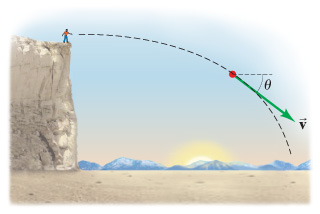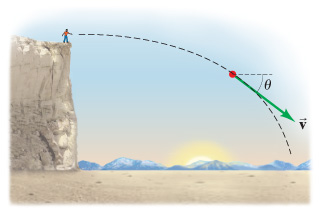# Problem: A ball is thrown horizontally from the top of a cliff with initial speed v0 (at t = 0). At any moment, its direction of motion makes an angle θ heta to the horizontal. Derive a formula for θ heta as a function of time, texttip{t}{t}, as the ball follows a projectile path.

###### FREE Expert Solution
80% (387 ratings)
###### FREE Expert Solution

In this problem, we apply the concepts in projectile motion to describe the motion of a projectile.

Uniform accelerated motion (UAM) equations, a.k.a. "kinematics equations":

80% (387 ratings)###### Problem Details

A ball is thrown horizontally from the top of a cliff with initial speed v0 (at t = 0). At any moment, its direction of motion makes an angle θ to the horizontal. Derive a formula for θ as a function of time, t, as the ball follows a projectile path.Frequently Asked Questions

What scientific concept do you need to know in order to solve this problem?

Our tutors have indicated that to solve this problem you will need to apply the Projectile Motion: Horizontal & Negative Launch concept. If you need more Projectile Motion: Horizontal & Negative Launch practice, you can also practice Projectile Motion: Horizontal & Negative Launch practice problems.

What professor is this problem relevant for?

Based on our data, we think this problem is relevant for Professor Padron's class at VALENCIA.